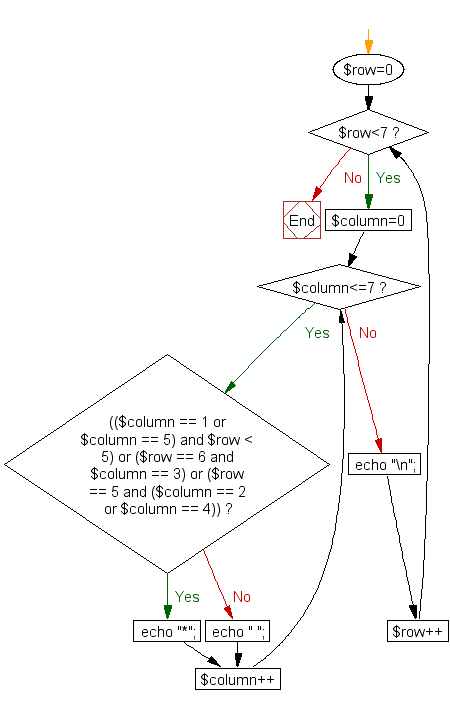﻿ PHP for loops Exercise: Print alphabet pattern V - w3resource# PHP for loop Exercises: Print alphabet pattern V

## PHP for loop: Exercise-34 with Solution

Write a PHP program to print alphabet pattern 'V'.

Pictorial Presentation:Sample Solution:

PHP Code:

``````<?php
for (\$row=0; \$row<7; \$row++)
{
for (\$column=0; \$column<=7; \$column++)
{
if (((\$column == 1 or \$column == 5) and \$row < 5) or (\$row == 6 and \$column == 3) or (\$row == 5 and (\$column == 2 or \$column == 4)))
echo "*";
else
echo " ";
}
echo "\n";
}
?>
```
```

Sample Output:

``` *   *
*   *
*   *
*   *
*   *
* *
*
```

Flowchart :PHP Code Editor:

Have another way to solve this solution? Contribute your code (and comments) through Disqus.

What is the difficulty level of this exercise?

﻿

New Content: Composer: Dependency manager for PHP, R Programming